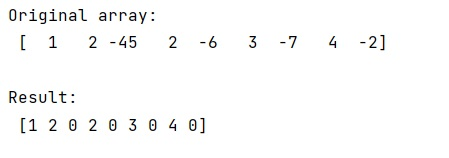# Replace negative values in a numpy array

Learn, how to replace negative values in a numpy array in Python?
Submitted by Pranit Sharma, on January 08, 2023

NumPy is an abbreviated form of Numerical Python. It is used for different types of scientific operations in python. Numpy is a vast library in python which is used for almost every kind of scientific or mathematical operation. It is itself an array which is a collection of various methods and functions for processing the arrays.

## NumPy Array - Replacing negative values

Suppose that we are given a NumPy array that contains negative values and we need to replace these negative values with 0.

For this purpose, we will use the indexing method where we will pass a specific condition that if a value is less than 0 then we will replace it with 0.

Let us understand with the help of an example,

## Python code to replace negative values in a numpy array

```# Import numpy
import numpy as np

# Import pandas
import pandas as pd

# Creating an array
arr = np.array([1,2,-45,2,-6,3,-7,4,-2])

# Display array
print("Original array:\n",arr,"\n")

# Replacing negative values
arr[arr < 0] = 0

# Display result
print("Result:\n",arr,"\n")
```

Output: